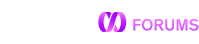# Python Pipeline Transform from HDFS

Hi,

Im trying to create a pipeline that will do the following:

• Read HDFS Folder with multiple JSON Files
• Use a pipeline to continously read new files
• Include in the pipeline a transform python script (that will transform the json to python pandas do some transformation and keep only the necessary columns)
• Load into a MemSQL Columnar Table

Currently my pipeline works withouth the tranform, this is the python script I made:

``````#!/usr/bin/python

import struct
import sys
from datetime import date, datetime
import time, json, ijson
import pandas as pd
from pandas.io.json import json_normalize

binary_stdin = sys.stdin if sys.version_info < (3, 0) else sys.stdin.buffer
binary_stderr = sys.stderr if sys.version_info < (3, 0) else sys.stderr.buffer
binary_stdout = sys.stdout if sys.version_info < (3, 0) else sys.stdout.buffer

def input_stream():
"""
Consume STDIN and yield each record that is received from MemSQL
"""
while True:
if len(byte_len) == 8:
byte_len = struct.unpack("L", byte_len)
yield result
else:
assert len(byte_len) == 0, byte_len
return

def log(message):
"""
Log an informational message to stderr which will show up in MemSQL in
the event of transform failure.
"""
binary_stderr.write(message + b"\n")

def transformToCSV(file):
with open(file) as jsonFile:
dataNormal = json_normalize(data, sep='_')
df = pd.DataFrame(dataNormal)
df['timestamp'] = pd.to_datetime(df['timestamp'])
df['date'] = pd.to_datetime(df['timestamp']).dt.date
df = df[["metric_id","timestamp","date","service","type",
"scene","name","observation","observation"]]
dfFinal = df.to_csv(df, encoding="utf-8",index=False)
binary_stdout.write(dfFinal)

log(b"Begin transform")

# We start the transform here by reading from the input_stream() iterator.
for data in input_stream():
# Since this is an identity transform we just emit what we receive.
transformToCSV(data)

log(b"End transform")
``````

But when I test the pipeline I get the following error:
ERROR: ImportedOS::ExecuteSubprocessAsync()

(Pipeline without the transform works just fine)

What I am missing? I couldn’t find any example of using pipelines transform with hdfs data.

Thanks,

This transform looks like its trying to use the kafka transform protocol. The HDFS (and S3 and FS and Azure) transform protocol is actually much simpler, it just receives the file on stdin and writes the output to stdout. Thus you don’t need the `input_stream` function at all.

Hope this helps,

Also, its worth noting that we have direct JSON ingest in 6.7 and later, so you don’t even need to convert to CSV. https://docs.memsql.com/sql-reference/v6.7/load-data/. You do, in 6.7, need to say what values in the json you output map to what values in your table, but once you’ve constructed a dict (call it `obj`) in python, you can simply do `print json.dumps(obj)` and it to emit the record.

1 Like

If it’s possible to express your data transformations as SQL expressions, I’d also recommend transforming data using CREATE PIPELINE’s optional SET clause and omitting the TRANSFORM clause from CREATE PIPELINE entirely. Piping data through any transform has overhead that’s worth avoiding. And in your particular case, even if the transform outputs JSON instead of CSV, it’ll still involve parsing JSON in python.

As I don’t need the input_stream function at all, could I get a simple example of a transfrom without it?

Could be something like this?:

``````def transformToCSV(sourceFile):
with open(sourceFile) as jsonFile:
df = pd.DataFrame(data)
finalDF = df.to_csv("output.csv", encoding="utf-8", index=False)
sys.stdout.write(finalDF)

transformToCSV(sys.stdin)
``````

I ask for a simple example because we don’t only want to open the JSON/csv file directly from HDFS and load everything to MemSQL. Based on different scenarios we might do some simple calculations and upload results to a table. (Thus, we need to open the json file, do something with it in python and save it in the table)

Thanks again,

Hi,

Did you created a python function to add transformation in which we can add new columns?
Source file can be csv and target table will have an extra column(which we will add in python code of transformation )

if you did please share. It would be a great help.

what I want to do:
want to add new column to the data coming from the pipeline and insert it into the table along with new column. Of course my table schema already contains new column that will be added.

Thanks in advance